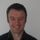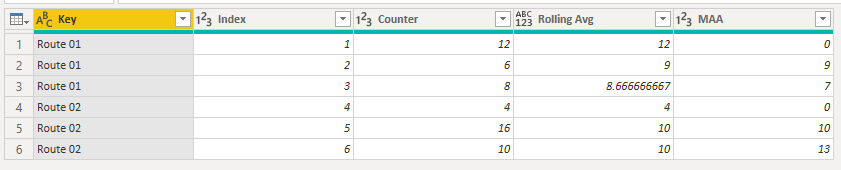cancel
Showing results for
Did you mean:

Fabric is Generally Available. Browse Fabric Presentations. Work towards your Fabric certification with the Cloud Skills Challenge.Resolver I

## Grouped Rolling Average

Hi

I have the following m code to create a grouped running average:

try

let Group=[Route_DU_Key], Row=[Index] in
List.Average(Table.SelectRows(#"Added Index", each [Index]<=Row and [Route_DU_Key]=Group)[Counter])

otherwise 0

And the following m code to create a 13 period rolling average:

I want to create a grouped, 13 period rolling average, but am going round and round and keep getting errors when I try to combine them. Can anyone help with this? Thanks.

2 ACCEPTED SOLUTIONSSuper User

Hi @pbix1

This M code creates a rolling average based on the sample data you supplied.  It does not need an Index column to work.

It will work with any number of 'Keys'.``````let

GRAList = (values as list, grouping as list) as list =>

let

TheList = List.Generate
(
()=> [ GRT = values{0}, GRA = values{0}, i = 0, j = 1 ],

each [i] < List.Count(values),

each try
if grouping{[i]} = grouping{[i] + 1} then [GRT = [GRT] + values{[i]+1} , GRA = GRT/j, i = [i] + 1, j = [j] + 1]

else [GRT = values{[i]+1} , GRA = GRT/j, i = [i] + 1, j = 1]

otherwise [i = [i] + 1],

each [GRA]
)
in
TheList,

Source = Table.FromRows(Json.Document(Binary.Decompress(Binary.FromText("i45WCsovLUlVMDBU0lECYyMgYaAUq4MiAxI0A2JLdAljILYAYnNkCZBqEyg2QJcwBVkCMssQQwoqCiSMlWJjAQ==", BinaryEncoding.Base64), Compression.Deflate)), let _t = ((type nullable text) meta [Serialized.Text = true]) in type table [Key = _t, Index = _t, Counter = _t, MAA = _t]),
#"Changed Type" = Table.TransformColumnTypes(Source,{{"Index", Int64.Type}, {"Counter", Int64.Type}, {"MAA", Int64.Type}}),

BufferedValues = List.Buffer(#"Changed Type"[Counter]),
BufferedGrouping = List.Buffer(#"Changed Type"[Key]),

GroupedRunningAverage = Table.FromList(GRAList(BufferedValues, BufferedGrouping), Splitter.SplitByNothing(), {"GRA"}, null, ExtraValues.Error),
Columns = List.Combine({Table.ToColumns(#"Changed Type"),Table.ToColumns(GroupedRunningAverage)}),
#"Converted to Table" = Table.FromColumns(Columns,List.Combine({Table.ColumnNames(#"Changed Type"),{"Rolling Avg"}})),
#"Reordered Columns" = Table.ReorderColumns(#"Converted to Table",{"Key", "Index", "Counter", "Rolling Avg", "MAA"})
in
#"Reordered Columns"``````

Regards

Phil

If my answer helped solve your problem, give it a kudos by clicking on the Thumbs Up.

If I helped you, click on the Thumbs Up to give Kudos.

Proud to be a Super User!Resolver I

Hi. Thanks for all the responses.  Phil, I tried your method but I could only see how it worked for a rolling average which I had already had a method for. I was after a rolling average by group. I found this method, which after a bit of adapting, worked for what I needed. Link below in case it helps anyone else:

https://stackoverflow.com/questions/55337952/power-query-m-language-50-day-moving-average

Finally! found a solution. First, apply Index by product see this post for further details Then index again without criteria (index all rows) Then, apply below code
= Table.AddColumn(#"Previous Step", "Volume SMA(50)", each if [Index_byProduct] >= 50 then List.Average(List.Range(#"Previous Step"[Volume], ([Index_All]-50),50)) else 0),
For large dataset, Table.Buffer function is recommended after index-expand step to improve PQ calculation speed

7 REPLIES 7Super User

Hi @pbix1

This M code creates a rolling average based on the sample data you supplied.  It does not need an Index column to work.

It will work with any number of 'Keys'.``````let

GRAList = (values as list, grouping as list) as list =>

let

TheList = List.Generate
(
()=> [ GRT = values{0}, GRA = values{0}, i = 0, j = 1 ],

each [i] < List.Count(values),

each try
if grouping{[i]} = grouping{[i] + 1} then [GRT = [GRT] + values{[i]+1} , GRA = GRT/j, i = [i] + 1, j = [j] + 1]

else [GRT = values{[i]+1} , GRA = GRT/j, i = [i] + 1, j = 1]

otherwise [i = [i] + 1],

each [GRA]
)
in
TheList,

Source = Table.FromRows(Json.Document(Binary.Decompress(Binary.FromText("i45WCsovLUlVMDBU0lECYyMgYaAUq4MiAxI0A2JLdAljILYAYnNkCZBqEyg2QJcwBVkCMssQQwoqCiSMlWJjAQ==", BinaryEncoding.Base64), Compression.Deflate)), let _t = ((type nullable text) meta [Serialized.Text = true]) in type table [Key = _t, Index = _t, Counter = _t, MAA = _t]),
#"Changed Type" = Table.TransformColumnTypes(Source,{{"Index", Int64.Type}, {"Counter", Int64.Type}, {"MAA", Int64.Type}}),

BufferedValues = List.Buffer(#"Changed Type"[Counter]),
BufferedGrouping = List.Buffer(#"Changed Type"[Key]),

GroupedRunningAverage = Table.FromList(GRAList(BufferedValues, BufferedGrouping), Splitter.SplitByNothing(), {"GRA"}, null, ExtraValues.Error),
Columns = List.Combine({Table.ToColumns(#"Changed Type"),Table.ToColumns(GroupedRunningAverage)}),
#"Converted to Table" = Table.FromColumns(Columns,List.Combine({Table.ColumnNames(#"Changed Type"),{"Rolling Avg"}})),
#"Reordered Columns" = Table.ReorderColumns(#"Converted to Table",{"Key", "Index", "Counter", "Rolling Avg", "MAA"})
in
#"Reordered Columns"``````

Regards

Phil

If my answer helped solve your problem, give it a kudos by clicking on the Thumbs Up.

If I helped you, click on the Thumbs Up to give Kudos.

Proud to be a Super User!Resolver I

Hi. Thanks for all the responses.  Phil, I tried your method but I could only see how it worked for a rolling average which I had already had a method for. I was after a rolling average by group. I found this method, which after a bit of adapting, worked for what I needed. Link below in case it helps anyone else:

https://stackoverflow.com/questions/55337952/power-query-m-language-50-day-moving-average

Finally! found a solution. First, apply Index by product see this post for further details Then index again without criteria (index all rows) Then, apply below code
= Table.AddColumn(#"Previous Step", "Volume SMA(50)", each if [Index_byProduct] >= 50 then List.Average(List.Range(#"Previous Step"[Volume], ([Index_All]-50),50)) else 0),
For large dataset, Table.Buffer function is recommended after index-expand step to improve PQ calculation speedCommunity Champion

Hi @pbix1

Can you create a sample data and add the results that you expecting?

Best Regards,
Mariusz

If this post helps, then please consider Accepting it as the solution.

Please feel free to connect with me.Resolver I

Hi Mariusz

I'm trying to create a moving average that resets at each change of 'Key'. Where it produces an error because there are not enough periods to create it, this can show as zero. I have based this on a 2 period rolling average but would like the flexibility to change the number of periods.

Key                    Index                Counter            MAA

Route 01           1                       12                      0
Route 01           2                        6                       9
Route 01           3                        8                       7
Route 02           4                        4                       0
Route 02           5                        16                     10
Route 02           6                        10                     13

Does this help? Many thanks.

NeilMicrosoft

Here is a column expression that gets your desired results (although it does not give zero for the first value of a Key).

MAA =
VAR thisindex = Counter[Index]
VAR lasttwoindex =
TOPN (
2,
CALCULATETABLE (
VALUES ( Counter[Index] ),
ALLEXCEPT (
Counter,
Counter[Key]
),
Counter[Index] <= thisindex
),
Counter[Index], DESC
)
RETURN
CALCULATE (
AVERAGE ( Counter[Counter] ),
ALLEXCEPT (
Counter,
Counter[Key]
),
lasttwoindex
)

Regards,

Pat

Did I answer your question? Mark my post as a solution! Kudos are also appreciated!Resolver I

Hi Pat

Thanks for your reply. That looks like DAX though. I'm after a solution in m code to use in Power Query. I'm not really comfortable with DAX, and as an MS Access user I find Power Query a lot easier to understand.

Thanks - NeilCommunity Champion

Hi @pbix1

Please see the below, however, DAX calculation would probably be a better solution for this sort of manipulations.

``````let
Source = Table.FromRows(Json.Document(Binary.Decompress(Binary.FromText("i45WCsovLUlVMDBU0lECYyMgYaAUq4MiAxI0A2JLdAljILYAYnNkCZBqEyg2QJcwBVkCMssQQwoqCiSMlWJjAQ==", BinaryEncoding.Base64), Compression.Deflate)), let _t = ((type nullable text) meta [Serialized.Text = true]) in type table [Key = _t, Index = _t, Counter = _t, MAA = _t]),
#"Changed Type" = Table.TransformColumnTypes(Source,{{"Key", type text}, {"Index", Int64.Type}, {"Counter", Int64.Type}, {"MAA", Int64.Type}}),
#"Grouped Rows" = Table.Group(#"Changed Type", {"Key"}, {{"rows", each _, type table [Key=nullable text, Index=nullable number, Counter=nullable number, MAA=nullable number]}}),
#"Expanded Index" = Table.ExpandListColumn(#"Added Custom", "Index"),
#"Changed Type1" = Table.TransformColumnTypes(#"Expanded Index",{{"Index", Int64.Type}}),
let
lesThanIndex = Table.SelectRows( [rows], (a) =>  a[Index] <= [Index] ),
sort = Table.Sort(lesThanIndex,{{"Index", Order.Descending}}),
namberOfPeriods = 2,
FirstN =  Table.FirstN( sort, namberOfPeriods )[Counter]
in
if List.NonNullCount(  FirstN ) = namberOfPeriods then List.Average( FirstN ) else 0
)
in

Best Regards,
Mariusz

If this post helps, then please consider Accepting it as the solution.

Please feel free to connect with me.Announcements#### Power BI Monthly Update - November 2023

Check out the November 2023 Power BI update to learn about new features.#### Fabric Community News unified experience

Read the latest Fabric Community announcements, including updates on Power BI, Synapse, Data Factory and Data Activator.#### Exclusive opportunity for Women!

Join us for a free, hands-on Microsoft workshop led by women trainers for women where you will learn how to build a Dashboard in a Day!#### The largest Power BI and Fabric virtual conference

130+ sessions, 130+ speakers, Product managers, MVPs, and experts. All about Power BI and Fabric. Attend online or watch the recordings.Top Solution Authors
Top Kudoed Authors
Users online (4,018)